Pages created and updated by Terry Sturtevant Date Posted: May 12, 2017

# Maple Logic Tutorial

Maple is a commercial program. It is a computer algebra system (CAS) like Maxima or Mathematica.
There are various tutorials out there on how to use Maple; this one is designed to focus on its use for digital circuit analysis; i.e. lots of use of Boolean algebra.

## Sample Digital Logic Circuit

Here is a simple circuit:
Prime Number Indentifier Circuit

It gives us the following boolean Sum-Of-Products (SOP) equation :

prime = a3 a2 a1 + a3 a2 a0 + a2 a1 a0 + a2 a1 a0

A computer algebra system can be very useful for analyzing circuits like this.

6. ### Redefining Variables

1. Opening Maple:
• Open Maple from the menu or from its icon on the desktop.

2. Entering Equations:
•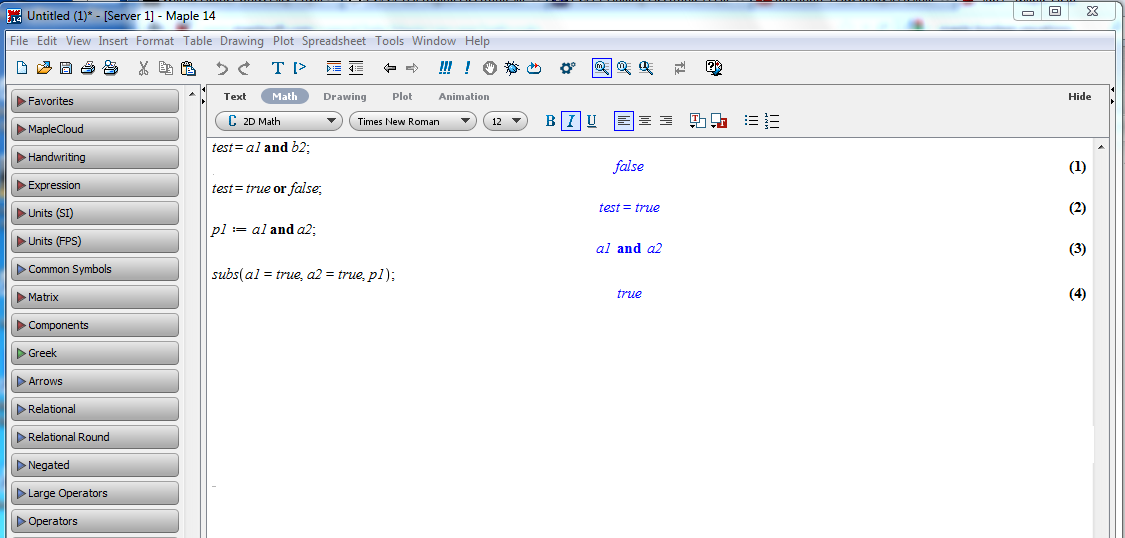To enter a boolean equation,
At the prompt, type in the the command as shown:
```  t1:= (not a3) and (not a2 and a1);
(not a3) and (not a2) and a1

```

This is the first term in the equation; we can type in each term separately to keep them all straight.
``` t2:= (not a3) and (a2 and a0);
(not a3) and a2 and a0
t3:=(not a2) and (a1 and a0);
(not a2) and a1 and a0
t4:=(not a1) and (a2 and a0);
(not a1) and a2 and a0
```

We can combine the individual terms easily by their names, like this:
```  result:= t1 or t2 or t3 or t4;
((not a3) and (not a2) and a1) or ((not a3) and a2 and a0)
or ((not a2) and a1 and a0) or ((not a1) and a2 and a0)
```

Now result represents the complete expression for the function.
• You can substitute in specific component values:
``` subs( a0=false, a1=false,a2=false, a3=false,result);
false
```

• You can repeat the previous instruction with each of the different input possibilities to check the entire function.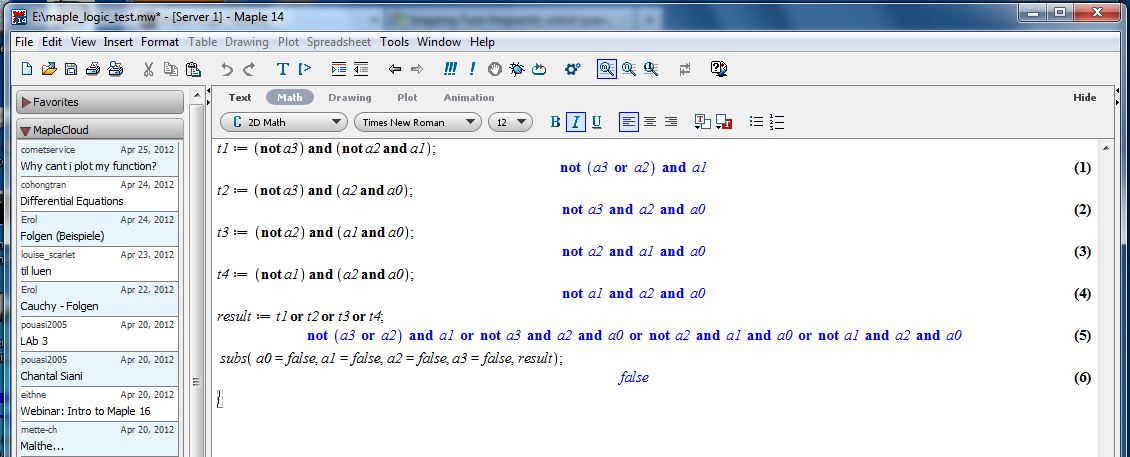• You can print the session for future reference as well..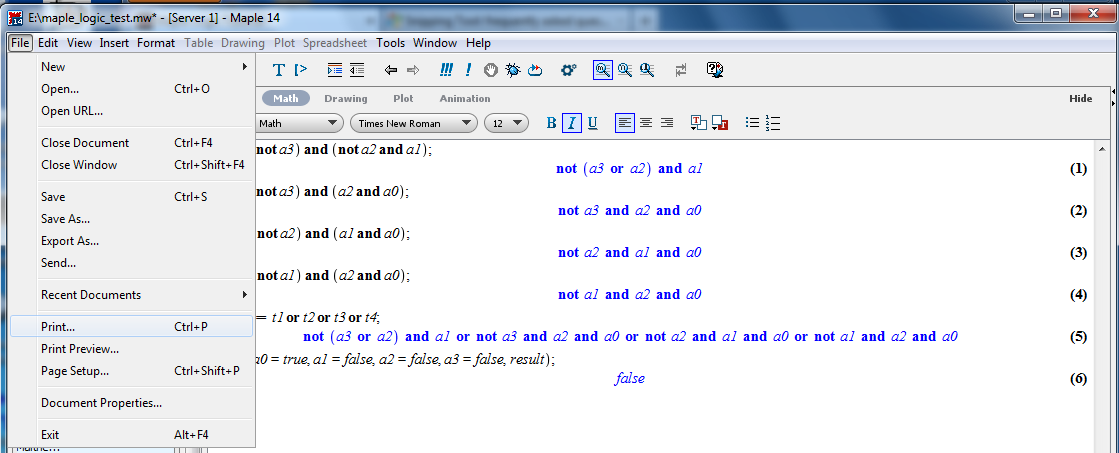3. Saving a Session:
• Saving a session: From the menu, choose the option to save.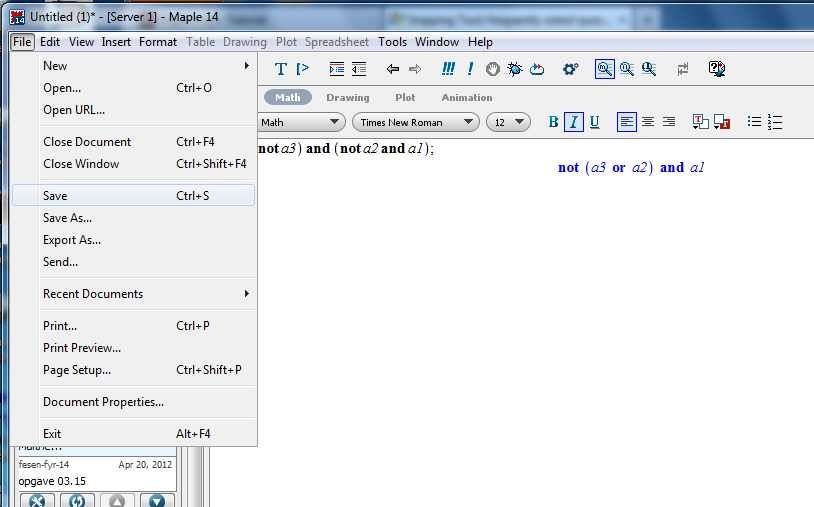4. Quitting Maple:
• Quitting maple: Choose Exit from the menu.

• It's nice to be able to pick up where you left off, so you can keep developing an analysis over time.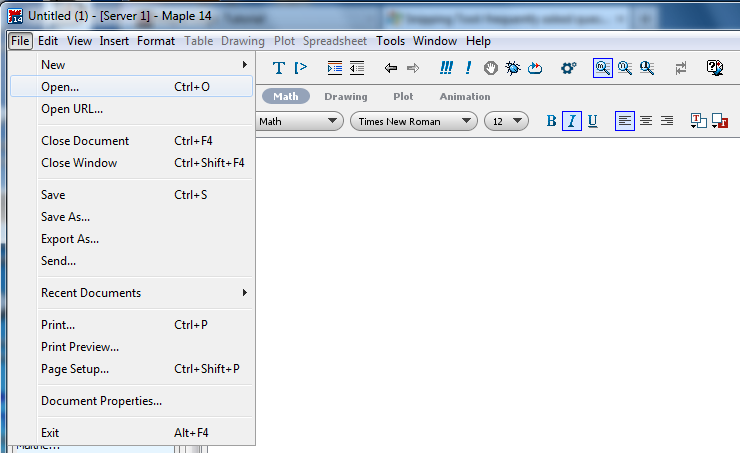Everything should be just as you left it.

• Previous statements can be re-executed and/or altered.

6. Redefining Variables:
• Variables can be changed by simply editing the lines on which they are defined and hitting Enter again.

We can continue on, substituting all of the input variable combinations.## Resources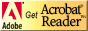If you need to update a browser, you might try Firefox which is
• free
• open source
• available for several platformsSince this page uses cascading style sheets for its layout, it will look best with a browser which supports the specifications as fully as possible.

If you are looking for an office package, with a word processor, spreadsheet, etc., you might try LibreOffice which is
• free
• open source
• available for several platforms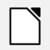Go to the main page for the Department of Physics and Computer Science.

Wilfrid Laurier University